Data Structures and Class Methods in scuff-em

This page is intended to serve as a starting point for hackers seeking to understand, or extend, the nitty-gritty implementation details of the scuff-em core library.

More technical details may be found in the libscuff Implementation Notes and Technical Reference, available as a PDF document.

Data Structures and Class Methods in scuff-em

1. Geometries, Regions, Surfaces
2. Panels, Edges, Vertices
3. Assembling the BEM Matrix
4. An Explicit Low-Level Example

1. Geometries, Regions, Surfaces

The top-level data structure in libscuff is a C++ class named RWGGeometry. The definition of this class is a little too big to present in full here (you can find it in the header file libscuff.h), but we will point out its most important data fields and class methods.

Geometries in scuff-em are represented a collection of two or more contiguous three-dimensional regions bounded by one or more two-dimensional surfaces. Material properties (permittivity and permeability) are homogeneous (spatially constant) in each region and described by a single scuff-em material description.

Each region is assigned an integer index starting from 0. The RWGGeometry includes the following data fields for identifying physical regions.

class RWGGeometry
{
...
int NumRegions;
char **RegionLabels;
MatProp **RegionMPs;
...
};

Here RegionLabels[i] is a string description for the ith region in the problem, and RegionMPs[i] is a pointer to an instance of MatProp describing its frequency-dependent material properies. (MatProp is a very simple class, implemented by the libmatprop submodule of scuff-em, for handling frequency-dependent material properties.)

RWGGeometry always starts off with a single region (region 0) with label Exterior and the material properties of vacuum. Each REGION statement in the .scuffgeo file then creates a new region, starting with region 1. (This is true unless the label specified to the REGION keyword is Exterior, in which case the statement just redefines the material properties of region 0.) Each OBJECT...ENDOBJECT section in the .scuffgeo file also creates a single new region (for the interior of the object).

Regions in a geometry are separated from one another by surfaces. Each surface is described by a C++ class named RWGSurface. The RWGGeometry class maintains an internal array of RWGSurfaces:

class RWGGeometry
{
...
int NumSurfaces;
RWGSurface **Surfaces;
...
};

Each OBJECT...ENDOBJECT or SURFACE...ENDSURFACE section in the .scuffgeo file adds a new RWGSurface structure to the geometry. (Note that, in scuff-em parlance, an "object" is just a special case of a surface in which the surface is closed.)

The RWGSurface class is again slightly too complicated to list in full here, but we will discuss its most salient fields and methods. Among these are the RegionIndices field:

class RWGSurface
{
...
int RegionIndices;
...
};

These two integers are the indices of the regions on the two sides of the surface. The first region (RegionIndex) is the positive region for the surface; this means that the electric and magnetic surface currents on the surface contribute to the fields in that region with a positive sign. The second region (RegionIndex) is the negative region; currents on the surface contribute to the fields in that region with a negative sign.

Another way to think of this is that the surface normal vector n points away from RegionIndex and into RegionIndex.

2. Panels, Edges, Vertices

The mesh describing each surface in a geometry is structure is analyzed into lists of vertices, triangular panels, and panel edges. Several internal data fields in the RWGSurface class are devoted to storing this information.

class RWGSurface
{
...
int NumVertices;
double *Vertices;

int NumPanels;
RWGPanel **Panels;

int NumEdges;
RWGEdge **Edges;
...

};

Here Vertices is an array of 3*NumVertices double values in which the cartesian coordinates of each vertex are stored one after another. Thus, the x, y, z coordinates of the nvth vertex are Vertices[3*nv+0], Vertices[3*nv+1], Vertices[3*nv+2].

Panels and Edges are arrays of pointers to specialized data structures for storing geometric data.

The RWGPanel and RWGEdge structures

The elemental data structure in the scuff-em geometry hierarchy is RWGPanel. Each instance of this structure describes a single triangular panel in the mesh discretization of an RWGSurface.

RWGPanel definition

typedef struct RWGPanel
{
int VI;            /* indices of vertices in Vertices array */
int EI;            /* indices of edges in Edges array */

double Centroid;   /* panel centroid */
double ZHat;       /* normal vector */
double Area;          /* panel area */

int Index;            /* index of this panel within RWGSurface */

} RWGPanel;

Here the elements of the VI array are the indices of the three panel vertices within the list of vertices for the given RWGSurface. The Index field in RWGPanel indicates that panel's index within the Panels array of the parent RWGSurface. The remaining fields tabulate some useful geometric data on the panel.

In addition to an RWGPanel structure for each triangle in the surface mesh, we also create an RWGEdge structure for each panel edge.

RWGEdge definition

typedef struct RWGEdge
{
int iV1, iV2, iQP, iQM;  /* indices of panel vertices (iV1&lt;iV2) */
double Centroid;      /* edge centroid */
double Length;           /* length of edge */

int iPPanel;             /* index of PPanel within RWGSurface (0..NumPanels-1)*/
int iMPanel;             /* index of MPanel within RWGSurface (0..NumPanels-1)*/
int PIndex;              /* index of this edge within PPanel (0..2)*/
int MIndex;              /* index of this edge within MPanel (0..2)*/
int Index;               /* index of this edge within RWGSurface (0..NumEdges-1)*/

RWGEdge *Next;           /* pointer to next edge in linked list */

} RWGEdge;

The iQP, iV1, iV2, iQM fields here are indices into the list of vertices for the parent RWGSurface. iV1 and iV2 are the actual endpoints of the edge. iQP and iQM denote respectively the current source and sink vertices for the RWG basis function corresponding to this edge.

iPPanel and iMPanel are the indices (into the Panels array of the parent RWGSurface) of the positive and negative panels associated with the edge. (The positive panel is the one from which the RWG current emanates; its vertices are iQP, iV1, iV2. The negative panel is the one into which the RWG current is sunk; its vertices are iQM, iV1, iV2.) The iPPanel and iMPanel fields take values between 0 and NumPanels-1, where NumPanels is defined in the parent RWGSurface.

PIndex and MIndex are the indices of the edge within the positive and negative RWGPanels. (The index of an edge within a panel is defined as the index within the panel of the panel vertex opposite that edge; the index of a vertex is its position in the VI array in the RWGPanel structure.)

The Index field of RWGEdge is the index of the structure within the Edges array of the parent RWGSurface.

The remaining fields store some geometric data on the edge. The Centroid field stores the cartesian coordinates of the midpoint of the line segment between vertices V1 and V2.

Here's an example of two panels (panel indices 17 and 39) in a surface mesh, and a single edge (edge index 24) shared between them. Panel 17 is the positive panel for the edge, while panel 39 is the negative panel for the edge; the corresponding direction of current flow is indicated by the arrows. The larger red numbers near the vertices are the indices of those vertices in the Vertices array. The smaller magenta and cyan numbers are the indices of the vertices within the VI arrays in the two RWGPanels. (The other four edges of this panel pair would also have corresponding RWGEdge structures; these are not shown in the figure.)3. Assembling the BEM Matrix

The RWGGeometry class contains a hierarchy of routines for assembling the BEM matrix. If you are simply using the code to solve scattering problems, you will only ever need to call the top-level routine AssembleBEMMatrix or perhaps the second-highest-level routine, AssembleBEMMatrixBlock. However, developers of surface-integral-equation methods may wish to access the lower-level routines for computing the interactions of individual RWG basis functions or for computing certain integrals over triangular regions.

AssembleBEMMatrix

The top-level matrix assembly routine is AssembleBEMMatrix. This routine loops over all unique pairs of RWGSurfaces in the geometry. For each unique pair of surfaces, the routine calls AssembleBEMMatrixBlock to assemble the subblock of the BEM matrix corresponding to a single pair of RWGSurfaces, then stamps this subblock into the appropriate place in the overall BEM matrix.

For example, if a geometry contains three RWGSurfaces, then its overall BEM matrix has the block structurewhere the $\mathbf{M}__{ij}$ subblock describes the interactions of surfaces i and j. In this case AssembleBEMMatrix proceeds by making 6 calls to AssembleBEMMatrixBlock, one for each of the diagonal and above-diagonal blocks. (The below-diagonal blocks are related to their above-diagonal counterparts by symmetry.)

AssembleBEMMatrixBlock

AssembleBEMMatrixBlock computes the subblock of the BEM matrix corresponding to a single pair of RWGSurfaces. For compact (non-periodic) geometries, this amounts to making just a single call to SurfaceSurfaceInteractions. For periodic geometries, this involves making multiple calls to SurfaceSurfaceInteractions in which one of the two surfaces is displaced through various lattice vectors L to account for the contribution of periodic images.

The contribution of the matrix subblock computed by SurfaceSurfaceInteractions with displacement vector L is weighted in the overall BEM matrix by a Bloch phase factor $e^{i\mathbf{k}\cdot \mathbf{L}}$ where k is the Bloch wavevector, i.e. we havewhere the L superscript indicates that the corresponding matrix subblock is to be computed with one of the two surfaces displaced through translation vector L.

SurfaceSurfaceInteractions

SurfaceSurfaceInteractions loops over all RWGEdge structures on each of the two surfaces it is considering. For each pair of edges, it calls EdgeEdgeInteractions to compute the inner products of the RWG basis functions with the G and C dyadic Green's functions for each of the material regions through which the two surfaces interact. (Surfaces may interact through 0, 1, or 2 material regions.) Then it stamps these values into their appropriate places in the BEM matrix subblock.

For example, if we have surfaces Sα and Sβ that interact through a single dielectric medium, the structure of the corresponding matrix subblock is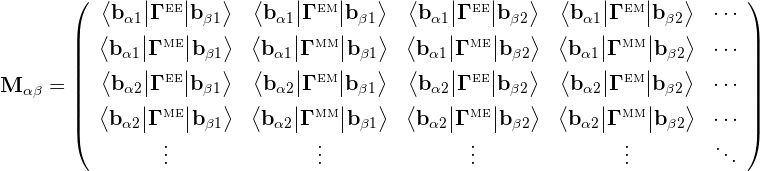where e.g. bαm is the mth basis function on surface Sα. The kernels here are scalar multiples of the G and C dyadics: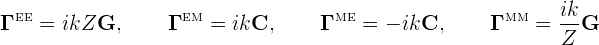where k, Z are the wavenumber and (absolute) wave impedance of the dielectric medium at the frequency in question.

The matrix structure above is for the case of two surfaces interacting through a single material region (for example, Sα and Sβ might be the outer surfaces of two compact objects embedded in vacuum or in a homogeneous medium, in which case the surfaces interact only throught that medium). If the surfaces interact through two material regions (for example, if we have Sα=Sβ and we are computing the self-interaction of the outer surface of a dielectric object embedded in vacuum) then each matrix entry is actually a sum of two inner products, one involving the Γ kernels for the interior medium and one involving the kernels for the exterior. (If the surfaces are PEC, then the dimension of the matrix is halved with only the EE terms retained.)

EdgeEdgeInteractions

EdgeEdgeInteractions considers a pair of RWG basis functions and computes the inner products of these basis functions with the G and C dyadic Green's functions for a single material medium. Because each basis function is supported on two triangles, the full inner products involve sums of four triangle-pair contributions:Here lm,ln are the lengths of the interior edges to which the RWG basis functions are associated, and each of the four terms in the sum is a four-dimensional integral over a single pair of panels, computed by PanelPanelInteractions.

PanelPanelInteractions

PanelPanelInteractions is the lowest-level routine in the scuff-em BEM matrix assembly hierarchy. This routine computes the individual terms in equation (1) above, i.e. the contributions of a single pair of panels to the inner products of two RWG basis functions with the G and C dyadic Green's functions: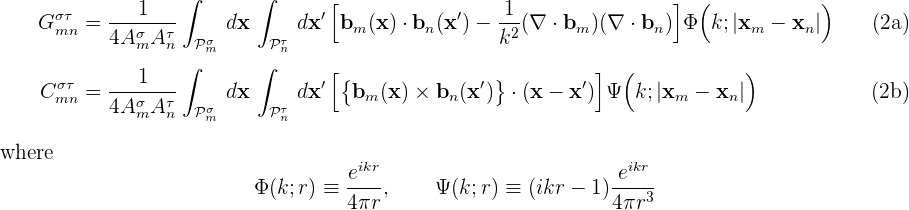Note that the RWG basis-function prefactor l/2A is broken up into two factors between equations (1) and (2): the 1/2A part is included in the panel-panel integrals (2), while the l part is included when summing the four panel-panel contributions in equation (1) to obtain the overall inner product.

If the panels are far away from each other, PanelPanelInteractions uses low-order four-dimensional numerical cubature to compute the full interactions. Otherwise, PanelPanelInteractions uses low-order four-dimensional numerical cubature to compute the interactions with singularity-subtracted versions of the G and C kernels, then adds the contributions of the singular terms after looking them up in an internally-stored cache.

4. An Explicit Low-Level Example

Here's a worked example of a matrix-element computation in a scuff-em run.

The geometry and the labeling of panels, edges, and vertices

We'll consider a scattering geometry consisting of a single cube of dielectric material, with side length L=1 μm, discretized into triangles with minor side length L/10, yielding a total of 1200 triangles, 1800 interior edges, and 3600 RWG basis functions (1800 each for electric and magnetic currents) for a dielectric geometry. The gmsh geometry file for this example is Cube_N.geo, the gmsh mesh file produced by running gmsh -2 Cube_N.geo is Cube_10.msh, and a scuff-em geometry file describing a geometry consisting of this discretized cube with interior dielectric permittivity ε=4 is DielectricCube.scuffgeo.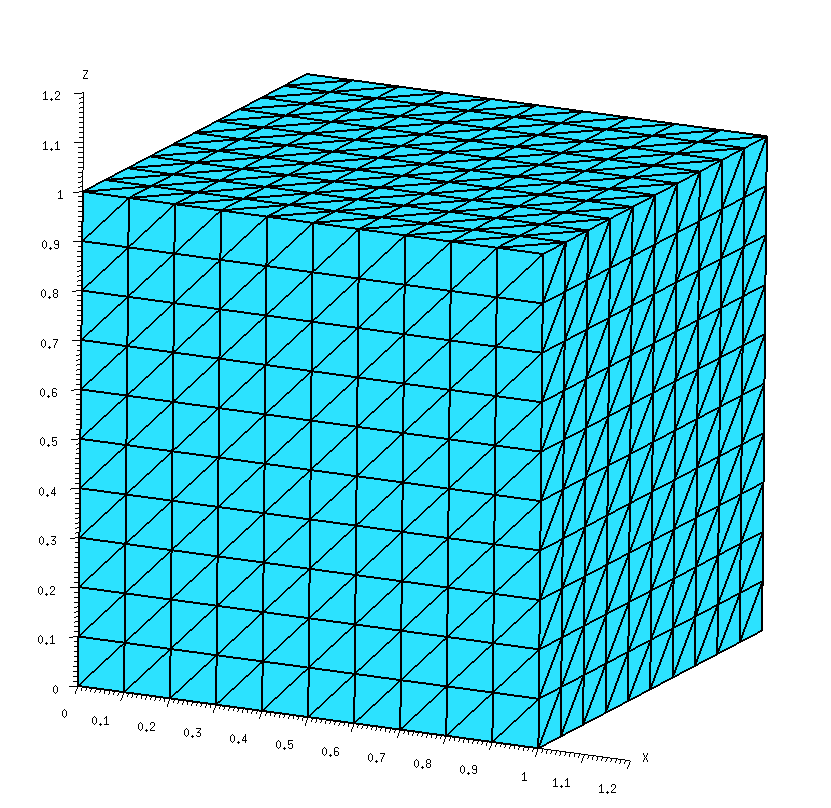For low-level work it is convenient to have data on the internal indices that scuff-em assigns to panels, vertices, and edges when it reads in a geometry file. We get this by running scuff-analyze on the mesh file in question with the --WriteGMSHLabels options:

% scuff-analyze --mesh Cube_10.msh --WriteGMSHFiles --WriteGMSHLabels

This produces a file named Cube_10.pp, which we open in gmsh to produce a graphical depiction of the labeling of panels, edges, and vertices. Zooming in on the region near the origin, we will focus on the two panels lying closest to the origin in the xy plane; we see that scuff-em has assigned these two panels panel indices 0 and 1, respectively, while the edge they share has been assigned interior-edge index 0.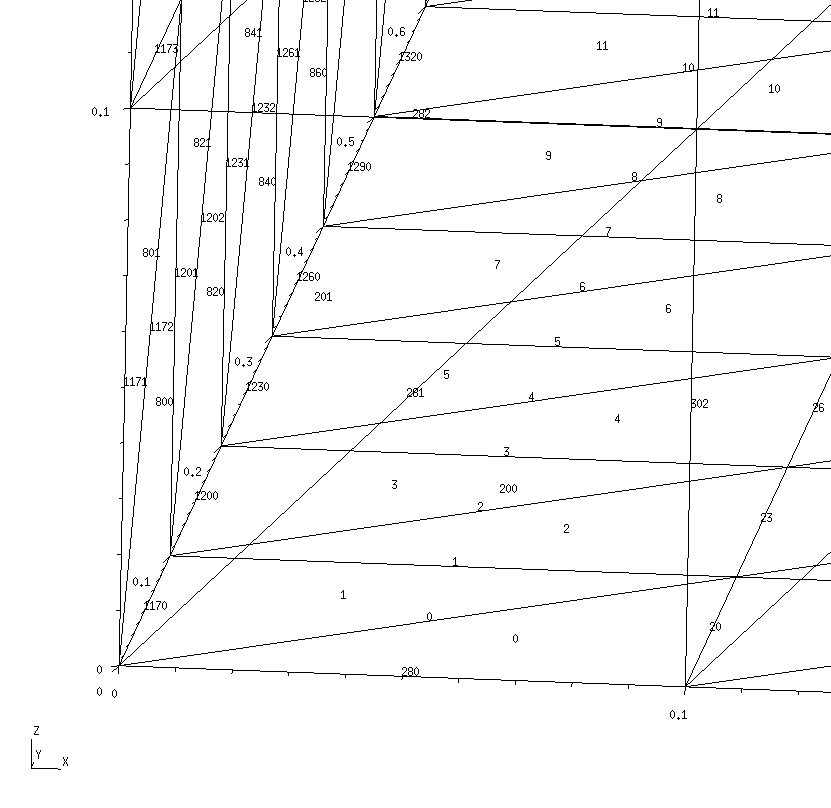RWG basis function b0

Here's a schematic depiction of the panels that comprise the basis function b0 associated with interior edge 0: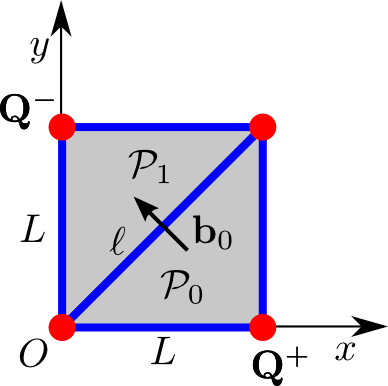In this diagram, the edge length is L=0.1, O denotes the origin of coordinates, and the vertices marked Q± are the source and sink vertices for the current distribution. The RWG basis function b0 associated with interior edge 0 is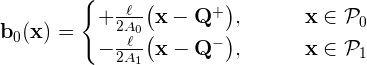where the RWG basis function edge length is l=√2L and the panel areas are A0=A1=L2/2. Note that P0 and P1 are respectively the positive and negative panels associated with basis function b0.

Panel-panel interactions

Here's a C++ code snippet that computes the quantities G00++ and G00+- in equation (1a) above, i.e. the contributions of the positive-positive and positive-negative panel pairs to the inner product of b0 with itself through the G kernel, with the wavenumber set to k=1.0; Just to be totally explicit, the numbers that are being computed here are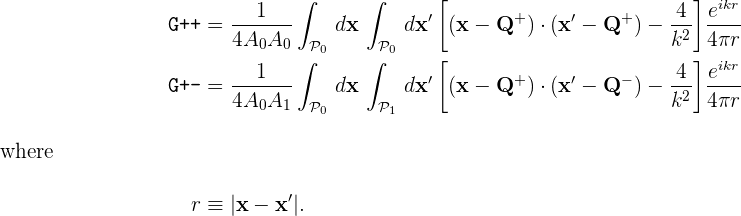// read in geometry from .scuffgeo file
RWGGeometry *G = new RWGGeometry("DielectricCube.scuffgeo");
RWGSurface *S = G->Surfaces;

// initialize an argument structure for GetPanelPanelInteractions
GetPPIArgStruct MyPPIArgs, *PPIArgs=&MyPPIArgs;
InitGetPPIArgs(PPIArgs);
PPIArgs->Sa = PPIArgs->Sb = S;
PPIArgs->k = 1.0;

// fill in arguments to compute the positive-positive panel pair
PPIArgs->npa = 0;
PPIArgs->iQa = 1;
PPIArgs->npb = 0;
PPIArgs->iQb = 1;
GetPanelPanelInteractions(PPIArgs);
printf("G++ = %e + %ei\n",real(PPIArgs->H),imag(PPIArgs->H));

// fill in arguments to compute the positive-negative panel pair
PPIArgs->npa = 0;
PPIArgs->iQa = 1;
PPIArgs->npb = 1;
PPIArgs->iQb = 2;
GetPanelPanelInteractions(PPIArgs);
printf("G++ = %e + %ei\n",real(PPIArgs->H),imag(PPIArgs->H));
This code produces the following output:
G++ = -3.189105e+00 + -7.950381e-02i
G+- = -1.537561e+00 + -7.956272e-02i

Notice that, to compute a panel-panel interaction, you allocate and initialize an instance of a data structure called GetPPIArgs. This structure contains a large number of fields which in many cases can be set to default values; to ensure that these fields are properly initialized, always call InitGetPPIArgs() on an newly-allocated instance of GetPPIArgs.

Then, you fill in the appropriate fields of this structure to specify the two panels over which you want to integrate. Specifically, the fields Sa, npa specify the first panel (the npath RWGPanel in the Panels array for surface Sa), while the field iQa (an integer in the range 0..2) identifies the index of the Q vertex (RWG current source/sink vertex) within the three vertices of the panel. The fields Sb, npb, and iQb similarly identify the second panel and source/sink vertex. Initialize the k field in the GetPPIArgs structure to the wavenumber parameter in the Helmholtz kernel. (k may be complex or purely imaginary.)

Edge-panel interactions

Here's a C++ code snippet that computes the full basis-function inner product < b0 | G | b0 > in equation (1a) above.

GetEEIArgStruct MyEEIArgs, *EEIArgs=&MyEEIArgs;
InitGetEEIArgs(EEIArgs);
EEIArgs->Sa = EEIArgs->Sb = S;
EEIArgs->nea = EEIArgs->neb = 0;
EEIArgs->k = 1.0;
GetEdgeEdgeInteractions(EEIArgs);
printf("&lt;b|G|b&gt; = %e + %ei\n",real(EEIArgs->GC),imag(EEIArgs->GC));

Note that, similar to the case of GetPanelPanelInteractions discussed above, the call to GetEdgeEdgeInteractions takes as argument a pointer to a struct of type GetEEIArgStruct. As before, you should always call InitGetEEIArgs to initialize this structure, then set whichever fields you need to specify. In this case, the only fields we need to set are Sa,Sb (indices of the RWGSurface), nea,neb (indices of the interior edges in the Edges array corresponding to the RWG basis functions), and k (wavenumber parameter in the Helmholtz kernel). The result of this code snippet is

<b|G|b> = -6.606176e-02 + 2.356554e-06i

Using equation (1a) above, we can understand this result in conjunction with the results printed out above for G++ and G+-. For this particular basis function, only two of the four panel-panel interactions on the RHS of equation (1a) have distinct values (because the two panels that comprise the basis function have the same shapes and areas, so we have G-+ = G+- and G-- = G++), and the
edge-length prefactors lm, ln both have value 0.1•√2. Thus for this case equation (1a) reads

< b0 | G | b0 > =2•0.02•(G++ - G+-)

and, indeed, plugging in the numbers printed out above, we find

{6.6e-02, 2.4e-06i} = 2•0.02•( {-3.19,-7.95e-2i} - {-1.54,7.96e-2i} ).